Win up to 100% scholarship on Aakash BYJU'S JEE/NEET courses with ABNAT Win up to 100% scholarship on Aakash BYJU'S JEE/NEET courses with ABNAT

# Algebra of Limits

The solution of equations and sets of equations is an essential and historically important part of what we call algebra. Algebra is commonly used in formulas when we can change one of the numbers or at least one of the numbers is unknown. In this article, you will learn the algebra of limits.

Algebra methods are used to evaluate the limits. Some of the important methods are the factorisation method, evaluation using standard limits, direct substitution method, rationalisation and evaluation of limits at infinity.

Let A and B be two functions such that their limits

$$\begin{array}{l}\lim_{x \rightarrow p} A(x)\ \text{and}\ \lim_{x \rightarrow p} B(x)\end{array}$$
exists, then the limit of the sum of two functions is the sum of the limits of the functions.

## Limit of Sum of Two Functions

Let A and B be two functions such that their limits

$$\begin{array}{l}\lim_{x \rightarrow p} A(x)\ \text{and}\ \lim_{x \rightarrow p} B(x)\ \text{exists, then}\end{array}$$
$$\begin{array}{l}\lim_{x \rightarrow p} A(x) \pm \lim_{x \rightarrow p} B(x)= \lim_{x \rightarrow p} (A \pm B)(x) \end{array}$$

Important Rules:

Let A(x) and B(x) be function of x such that

$$\begin{array}{l}\lim_{x \rightarrow p} A(x)=l\ \text{and}\ \lim_{x \rightarrow p} B(x)=m\end{array}$$

If l and m exist, then

(i) The sum/subtraction of the limits of two given functions is equal to the limit of the sum/subtraction of two functions.

$$\begin{array}{l}\lim_{x \rightarrow p}(A \pm B)(x)= \lim_{x \rightarrow p} A(x) \pm \lim_{x \rightarrow p} B(x) = l \pm m\end{array}$$

(ii) The limits of the product of any two given functions is the same as the product of the limits of that given function.

$$\begin{array}{l}\lim_{x \rightarrow p}(A \times B)(x)= \lim_{x \rightarrow p} A(x) \times \lim_{x \rightarrow p} B(x) = l \times m\end{array}$$

(iii) If we have the quotient of two functions, then the limit of that term is the same as the quotient of their limits, including the condition that the limit of the denominator is not equal to zero.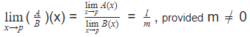(iv) The limit of a constant multiple of the function f(x) is equal to c times the limit of that function.

$$\begin{array}{l}\lim_{x \rightarrow p}k A(x)= k \lim_{x \rightarrow p}A(x);\ \text{where k is a constant.}\end{array}$$
$$\begin{array}{l}(v)\ \lim_{x \rightarrow p}| A(x)|=|\lim_{x \rightarrow p} A(x)|=|l|\end{array}$$
$$\begin{array}{l}(vi)\ \lim_{x \rightarrow p} A(x)^{B(x)}= \lim_{x \rightarrow p} A(x)^{\lim_{x \rightarrow p} B(x)} = l^m\end{array}$$
$$\begin{array}{l}(vii)\ \lim_{x \rightarrow p} AoB(x) = A(\lim_{x \rightarrow p}B(x)) = A(m),\end{array}$$
only if A(x) is continuous at B(x) = m

$$\begin{array}{l}(viii)\ \lim_{x \rightarrow p}= \infty\ \text{or}\ – \infty\ \text{then}\ \lim_{x \rightarrow p} \frac{1}{A(x)}= 0\end{array}$$

Important Points to Remember:

(i) limx→p (A.B)(x) exists, then we have the following cases:

(a) both limx→p A(x) and limx→p B(x) exists.

(b) limx→p A(x) exists, but limx→p B(x) does not exist.

Example: A(x) = x and B(x) = 1/x then limx→p A(x) = 0(exists) and limx→p B(x) does not exist, but limx→p (A.B)(x) = 1(exists)

(c) both limx→p A(x) and limx→p B(x) does not exist

Example: A(x) = 1 ∀ x ≥ 0 and 2 ∀ x < 0 and B(x) = 2 ∀ x ≥ 0 and 1 ∀ x < 0 then

limx→p A(x) does not exist and limx→p B(x) does not exist, but limx→p (A.B)(x) = 2(exists).

(ii) If limx→p [A(x)+B(x)] exists, then we have following cases:

(a) If limx→p A(x) exists, then limx→p B(x) must exist

Proof: This is true as B = [A + B] – A.

So, by the limit theorem, limx→p B(x) = limx→p [A(x) + B(x)] – limx→p B(x) which exists.

(b) Both limx→p A(x) and limx→p B(x) does not exist

Example: A(x) = [x], where [.] represents greatest integer function.

B(x) = {x}, where {.} represents the fraction part function.

Here, both the limits limx→p A(x) and limx→p B(x) do not exist, but limx→p [A(x) + B(x)] exists.

## Methods for Evaluating the Limits

(1) Direct substitution method:

(a) limx→p f(x)

(b) limx→p (A(x))/(B(x))

If f(a) and (A(a))/(B(a)) both exist and B(a)≠0, then limx→p f(x) = f(a) and limx→p (A(x))/(B(x))=(A(a))/(B(a)).

(2) Factorisation and rationalisation: Do the factorisation and rationalisation whenever needed.

(3) Evaluation of limits using standard substitution:

Some standards limits are given below:

• $$\begin{array}{l}\lim_{x \to a} \frac{x^{n}-a^{n}}{x-a}= n(a)^{n-1}\end{array}$$
• $$\begin{array}{l}\lim_{x \to 0} sin x = 0\end{array}$$
• $$\begin{array}{l}\lim_{x \to 0} cos x = 1\end{array}$$
• $$\begin{array}{l}\lim_{x \to 0} \frac{1-cos x}{x} = 0\end{array}$$
• $$\begin{array}{l}\lim_{x \to 0} \frac{sin^{-1}x}{x} = 1\end{array}$$
• $$\begin{array}{l}\lim_{x \to 0} \frac{tan^{-1} x}{x} = 1\end{array}$$
• $$\begin{array}{l}\lim_{x \to 0} \frac{sin x}{x} = 1\end{array}$$
• $$\begin{array}{l}\lim_{x \to 0} \frac{tan x}{x} = 1\end{array}$$
• $$\begin{array}{l}\lim_{x \to 0} e^{x}=1\end{array}$$
• $$\begin{array}{l}\lim_{x \to 0} \frac{e^{x}-1}{x}=1\end{array}$$
• $$\begin{array}{l}\lim_{x \to \infty } \left ( 1+\frac{1}{x} \right )^{x}=e\end{array}$$
• $$\begin{array}{l}\lim_{x \to \infty } \left ( 1+\frac{a}{x} \right )^{x}=e^{a}\end{array}$$
• $$\begin{array}{l}\lim_{x \to 0} (1+x)^{\frac{1}{x}}=e\end{array}$$
• $$\begin{array}{l}\lim_{x \to 0} \frac{a^{x}-1}{x}=log_{e}a\end{array}$$
• $$\begin{array}{l}\lim_{x \to 0} \frac{log(1+x)}{x}=1\end{array}$$

## Limits at Infinity and Infinite limits

The limit of a function shows the behaviour of the function at a particular point. The limit is the output value of the function for which the input value approaches closer and closer to a particular point. Let’s see, with the help of an example, how to compute infinity and infinite limits.

We know that

$$\begin{array}{l}\lim_{x \rightarrow +\infty}\frac{1}{x} = 0\end{array}$$
and
$$\begin{array}{l}\lim_{x \rightarrow \infty}\frac{1}{x^2} = 0\end{array}$$

So we have:

$$\begin{array}{l}\lim_{x \rightarrow +\infty}f(x) = \lim_{y \rightarrow 0} f\left(\frac{1}{y}\right)\end{array}$$

Example:

$$\begin{array}{l}\lim_{x \rightarrow \infty} \frac{\sqrt{5x^2+1}-\sqrt{7x^2-1}}{2x – 3}\end{array}$$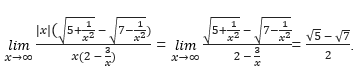## Sandwich Theorem

Sometimes we use the squeeze principle on limits where usual algebraic methods are not effective. In this method, adjust the given problem in between two other simpler functions whose limit can easily be computed and equal. Also, we need knowledge of algebra and inequalities.

If f, g and h are three functions such that f(x) < g(x) < h(x) for all x in some interval containing the point x = a, and if

$$\begin{array}{l}\lim_{x \rightarrow a}f(x) = \lim_{x \rightarrow a} h(x) = L\Rightarrow \lim_{x \rightarrow a}g(x) = L\end{array}$$

Example:

$$\begin{array}{l}\text{Compute}\ \lim_{x \rightarrow \infty}\frac{x – 5 sin x}{-5x – 7}\end{array}$$

Solution:

We know that range of sin x is [-1, 1], so min(sin x) = -1 and max(sin x) = 1

⇒ -1 ≤ sin x ≤ 1

⇒ -5 ≤ 5sin x ≤ 5

⇒ 5 ≥ -5. sin x ≥ -5

⇒ x + 5 ≥ x – 5. sin x ≥ x – 5

$$\begin{array}{l}\Rightarrow \frac{x – 5 sin x}{-5x – 7} \leq \frac{x – 5 sin x}{-5x – 7} \leq \frac{x – 5}{-5x – 7}\end{array}$$
$$\begin{array}{l}\lim_{x \rightarrow \infty}\frac{(x + 5)}{-5x – 7}\end{array}$$

= -1/5

So, we can say that

$$\begin{array}{l}\lim_{x \rightarrow \infty}\frac{x – 5 sin x}{-5x – 7}=-\frac{1}{5}\end{array}$$

### Practice Problems

1. $$\begin{array}{l}\text{Compute}\ \lim_{x \to 0} \frac{sin(2+x) – sin (2-x)}{x}\end{array}$$
2. Prove that limx→1 (6x + 1) = 7 by using the precise definition of a limit.
3. $$\begin{array}{l}\text{Find}\ \lim_{x\rightarrow0}\frac{sin(a+x)-sin(a-x)}{x}\end{array}$$

## Video Lessons

### Limits, Continuity and Differentiability – Part 1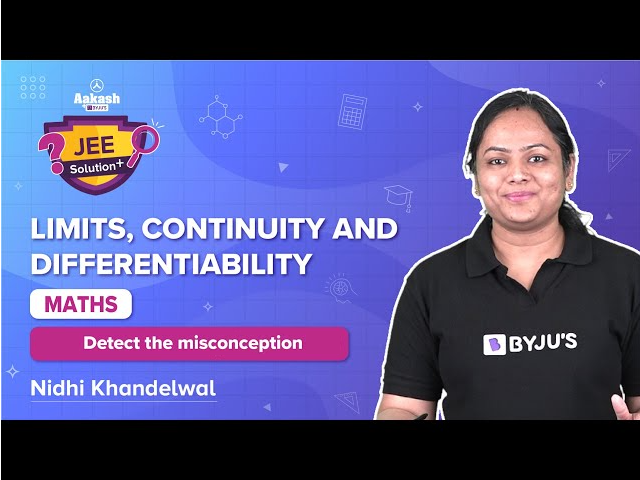### Limits, Continuity and Differentiability – Part 2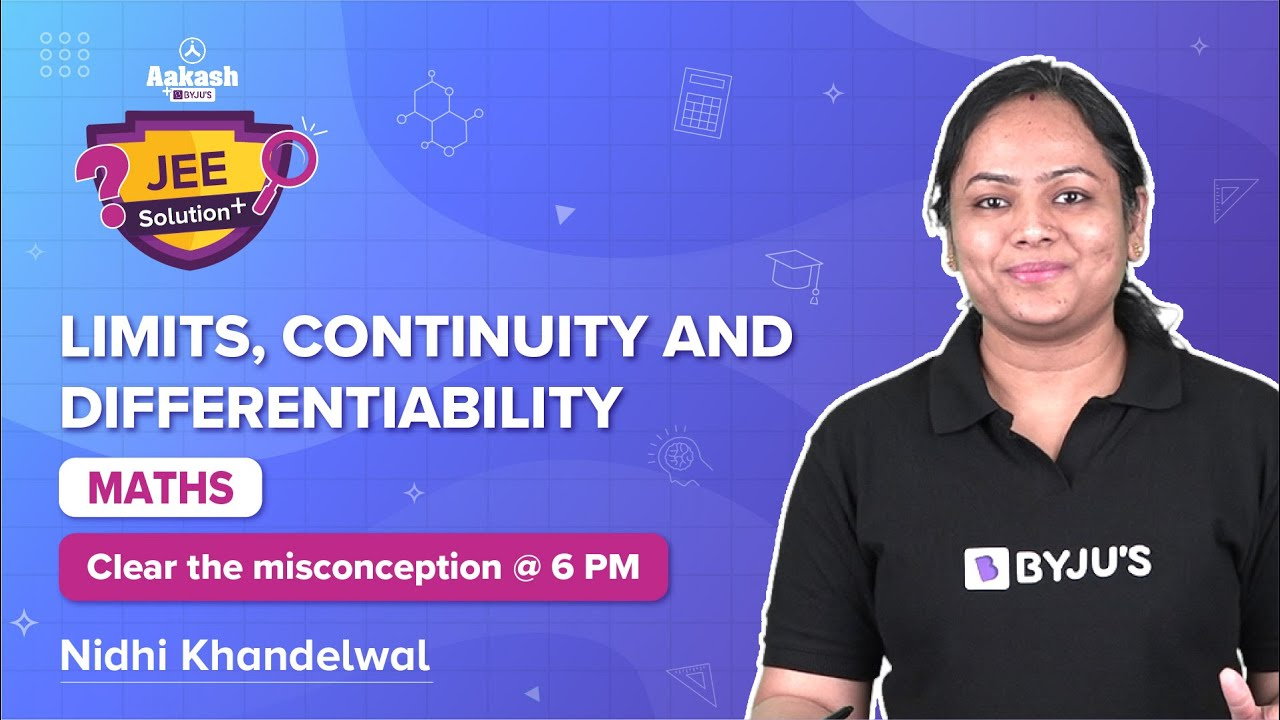### Limits, Continuity and Differentiability – Important Topics### Limits, Continuity and Differentiability – Important Questions### Limits for Class 11 – Revision Notes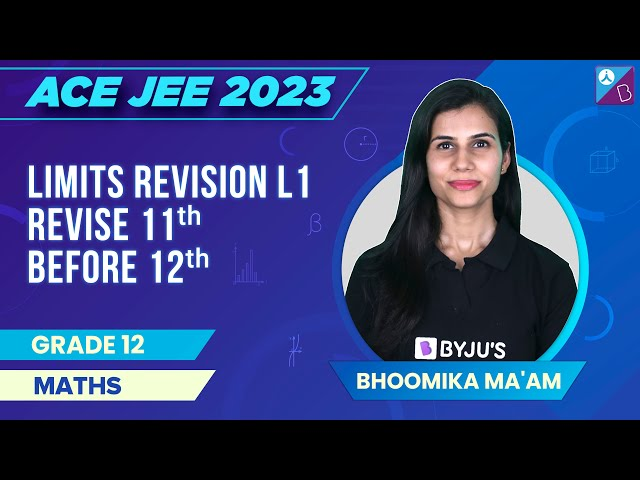Q1

### How do you find the sum of the limits of two functions?

The sum of the limits of two functions is equal to the limit of the sum of two functions.

Q2

### Mention the law of multiplication of limits.

The limits of the product of any two functions are the same as the product of the limits of the given functions. limx→a[f(x). g(x)] = limx→af(x).limx→ag(x).

Q3

### What is the value of lim x→0 (tan x)/x?

The value of lim x→0 (tan x)/x = 1.